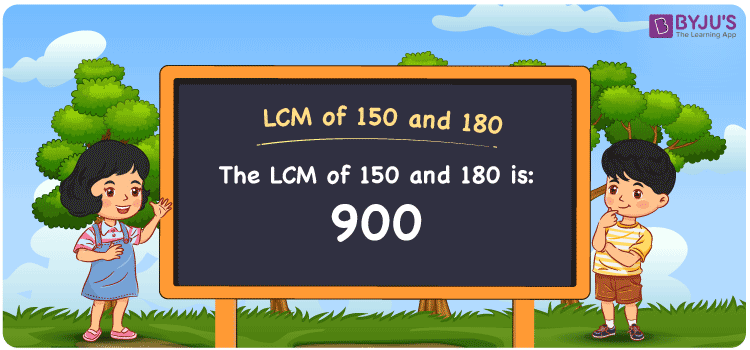# LCM of 150 and 180

LCM of 150 and 180 is 900. Get a good knowledge of the least common multiple of 150 and 180 referring to this article designed by the subject experts. The multiples of 150 are (150, 300, 450, 600, ….) and the multiples of 180 are (180, 360, 540, 720, 900, 1080,….). The LCM of two numbers according to prime factorisation, by listing out the multiples and division, is explained here in brief.

Also read: Least common multiple

## What is LCM of 150 and 180?

The answer to this question is 900. The LCM of 150 and 180 using various methods is shown in this article for your reference. The LCM of two non-zero integers, 150 and 180, is the smallest positive integer 900 which is divisible by both 150 and 180 with no remainder.## How to Find LCM of 150 and 180?

LCM of 150 and 180 can be found using three methods:

• Prime Factorisation
• Division method
• Listing the multiples

### LCM of 150 and 180 Using Prime Factorisation Method

The prime factorisation of 150 and 180, respectively, is given by:

150 = 2 x 3 x 5 x 5 = 2¹x 3¹ x 5²

180 = 2 x 2 x 3 x 3 x 5 = 2² x 3² x 5¹

LCM (150, 180) = 900

### LCM of 150 and 180 Using Division Method

We’ll divide the numbers (150, 180) by their prime factors to get the LCM of 150 and 180 using the division method (preferably common). The LCM of 150 and 180 is calculated by multiplying these divisors.

 2 150 180 2 75 90 3 75 45 3 25 15 5 25 5 5 5 1 x 1 1

No further division can be done.

Hence, LCM (150, 180) = 900

### LCM of 150 and 180 Using Listing the Multiples

To calculate the LCM of 150 and 180 by listing out the common multiples, list the multiples as shown below

 Multiples of 150 Multiples of 180 150 180 300 360 450 540 600 720 750 900 900 1080

The smallest common multiple of 150 and 180 is 900.

Therefore LCM (150, 180) = 900

## Video Lesson on Applications of LCM## LCM of 150 and 180 Solved Examples

Question: If the GCD = 30, LCM = 900 and one number is 150, determine the other.

Solution:

Consider z as the other number

GCD x LCM = 150 x z

z = (GCD x LCM)/150

z = (30 x 900)/150

z = 180

Hence, the LCM is 180.

## Frequently Asked Questions on LCM of 150 and 180

### What is the LCM of 150 and 180?

The LCM of 150 and 180 is 900.

### Write the methods used to get the LCM of 150 and 180.

The methods used to get the LCM of 150 and 180 are the Division Method, Prime Factorization Method and Listing multiples.

### Determine the GCF if the LCM of 150 and 180 is 900.

LCM x GCF = 150 x 180

Given

LCM of 150 and 180 = 900

900 x GCF = 27000

GCF = 27000/900 = 30

### Find the LCM of 150 and 180 with the help of prime factorisation.

With the help of prime factorisation

150 = 2 x 3 x 5 x 5

180 = 2 x 2 x 3 x 3 x 5

LCM of 150 and 180 is 900.

### Write the relation between GCF and LCM of 150 and 180.

The relation between GCF and LCM of 150 and 180 is

GCF x LCM = 150 x 180

GCF x LCM = 27000